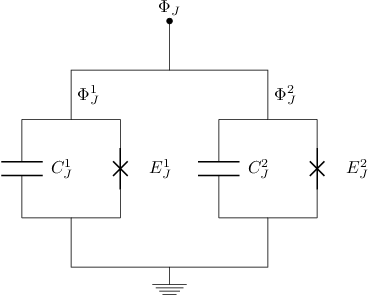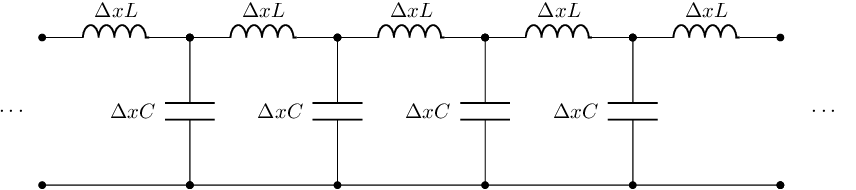IPython extension for drawing circuit diagrams with LaTeX/Circuitikz¶

Requirements¶

This IPython magic command uses the following external dependencies: pdflatex, pdfcrop, the Circuitikz package and

• for PNG output: convert from ImageMagick
• for SVG output: pdf2svg

Installation¶

In :
%install_ext http://raw.github.com/jrjohansson/ipython-circuitikz/master/circuitikz.py
Installed circuitikz.py. To use it, type:
/Users/rob/miniconda/lib/python2.7/site-packages/IPython/core/magics/extension.py:47: UserWarning: %install_ext` is deprecated, please distribute your extension(s)as a python packages.
"as a python packages.", UserWarning)

In :

Example: SQUID¶

In :
%%circuitikz filename=squid dpi=125

\begin{circuitikz}[scale=1]
\draw ( 0, 0) [short, *-] node[anchor=south] {$\Phi_J$} to (0, -1);

% right
\draw ( 0, -1) to (2, -1) to node[anchor=west] {$\Phi_{J}^2$} (2, -2) to (3, -2)
to [barrier, l=$E_J^2$] (3, -4) to (2, -4)to (2, -5) to (0, -5) node[ground] {};
\draw ( 2, -2) to (1, -2) to [capacitor, l=$C_J^2$] (1, -4) to (1, -4) to (2, -4);

% left
\draw ( 0, -1) to (-2, -1) to node[anchor=west] {$\Phi_{J}^1$} (-2, -2) to (-3, -2)
to [capacitor, l=$C_J^1$] (-3, -4) to (-2, -4)  to (-2, -5) to (0, -5);
\draw (-2, -2) to (-1, -2) to [barrier, l=$E_J^1$] (-1, -4) to (-1, -4) to (-2, -4);
\end{circuitikz}
Out:Example: Transmission line¶

In :
%%circuitikz filename=tm dpi=150

\begin{circuitikz}[scale=1.25]

\draw (-1,0) node[anchor=east] {} to [short, *-*] (1,0);
\draw (-1,2) node[anchor=east] {} to [inductor, *-*,  l=$\Delta x L$] (1,2);
\draw (-1,0) to [open, l=$\cdots$] (-1,2);
\draw (3, 0) to (1, 0) to [capacitor, l=$\Delta x C$, *-*] (1, 2) to [inductor, *-*, l=$\Delta x L$] (3, 2);
\draw (5, 0) to (3, 0) to [capacitor, l=$\Delta x C$, *-*] (3, 2) to [inductor, *-*, l=$\Delta x L$] (5, 2);
\draw (7, 0) to (5, 0) to [capacitor, l=$\Delta x C$, *-*] (5, 2) to [inductor, *-*, l=$\Delta x L$] (7, 2);
\draw (9, 0) to (7, 0) to [capacitor, l=$\Delta x C$, *-*] (7, 2) to [inductor, *-*, l=$\Delta x L$] (9, 2);
\draw (9,0) node[anchor=east] {} to [short, *-*] (9,0);
\draw (10,0) to [open, l=$\cdots$] (10,2);

\end{circuitikz}
Out: